# Mathematical Programming

Also found in: Financial.

(mathematics)

## Mathematical Programming

the mathematical discipline devoted to the theory and methods of finding the maxima and minima of functions on sets defined by linear and nonlinear constraints (equalities and inequalities).

Mathematical programming is a branch of operations research, encompassing a wide class of control problems, the mathematical models of which are finite-dimensional extremal problems. Mathematical programming problems are used in various fields of man’s activity where it is necessary to choose one course of action from several possible courses, for example, in the solution of the numerous problems of projection and of process control and planning.

The term “mathematical programming” reveals that the goal of the solution of these problems is the choice of a program of action.

The mathematical formulation of a mathematical programming problem is as follows: Minimize a scalar function φ(x) of the vector argument x on the set

X = {x:gi(x) ≥ 0, hi(x) = 0, i = 1, 2, …, k}

where gi(x) and hi(x) are also scalar functions. The function φ (x) is called the objective function, the set X is called the admissible set, and the solution x* of the mathematical programming problem is called the optimal point (vector).

In mathematical programming it is customary to distinguish linear, convex, quadratic, discrete, and stochastic programming. In linear programming the objective function φ(x) and the constraints gi(x) and hi(x) are linear, in convex programming the objective function and the admissible set are convex, and in quadratic programming the objective function is quadratic and convex and the admissible set is defined by linear equalities and inequalities. In discrete programming a solution is sought only at discrete— for example, integral — points of the set X. In stochastic programming, in contrast to a determinate problem, the input information contains elements of indeterminacy; for example, in stochastic problems of the minimization of the linear functionsubject to the linear constraints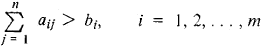either all the quantities cj, aij, bi or only some are random.

Problems in the branches of mathematical programming mentioned above possess the general property that every point of local minimum is an optimal point. Multi-extremal problems— problems for which the above property is not fulfilled—stand somewhat apart.

The theory of convex programming and, in particular, the theory of linear and quadratic programming are based on the Kuhn-Tucker theorem on the necessary and sufficient conditions for the existence of an optimal point x*: in order for the point x* to be optimal, that is,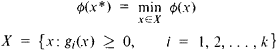it is necessary and sufficient that there exist a point y* = (y1*, y2*, …, yk*) such that the pair of points x*, y* form a saddle point of the Lagrange function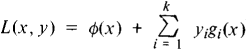The latter implies that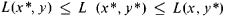for any x and all y ≥ 0. If the constraints gi(x) are nonlinear, then the theorem is valid for several additional assumptions regarding the admissible set.

If the functions (φ(x) and gi(x) are differentiable, then the following relations determine a saddle point: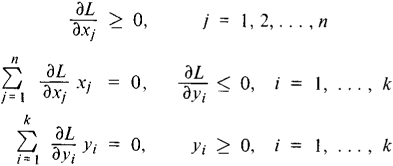Thus a problem in convex programming is reduced to the solution of a system of equations and inequalities.

On the basis of the Kuhn-Tucker theorem, various iterative methods of minimization have been developed that lead to the finding of a saddle point of the Lagrange function.

Computational methods for solving extremal problems occupy a principal place in mathematical programming. A broad class of such methods are the projection methods. The idea underlying these methods consists in the following. At the point xk ε X, we choose a direction of descent sk, that is, one of the directions along which the function φ(x) is decreasing, and we compute xk+1 = p(xk + aksk), where p(xk + aksk) is the projection of the point xk + akSk on the set X: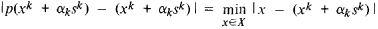where the number ak > 0 is chosen so that φ(xk+1) < φ(xk). There are various projection methods. The most common is the gradient projection method, where sk = — grad φ(k). In mathematical programming it has been proved that, under specific conditions for the objective function and the admissible set, the sequence (xk) constructed by the gradient projection method is such that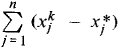approaches zero at the rate of a geometric progression .

The application of the methods of solving mathematical programming problems is inseparably connected with the use of computers. This is primarily because problems involving the control of actual systems are large-scale problems and cannot be computed manually.

An important area in mathematical programming research concerns problems of stability. Of essential importance is the study of stable problems; these are problems for which small perturbations (errors) in the input information entail small perturbations in the solution. In the case of unstable problems, an important role is assumed by the procedure of approximating an unstable problem by a sequence of stable problems— the method of regularization.

Mathematical programming as a science was formulated between the 1950’s and 1970’s. This was primarily dependent on the development of computers, and, consequently, on the possibility of mathematically processing large streams of data and thus solving control and planning problems, where the application of mathematical methods is mainly connected with the construction of mathematical models and their corresponding extremal problems, including mathematical programming problems.

### REFERENCES

Zukhovitskii, S. I, and L. I. Aveeva. Lineinoe i vypukloe programmirovanie, 2nd ed. Moscow, 1967.
Hadley, G. Nelineinoe i dinamicheskoe programmirovanie. Moscow, 1967. (Translated from English.)

V. G. KARMANOV

References in periodicals archive ?
Zhang: Higher order invexity and duality in mathematical programming, in Generalized Convexity, Generalized Monotonicity : Recent Results (J.
Generally speaking, there are three majors themes in the interplay of SVMs and mathematical programming. The first theme contains the development of under-lying models for standard classification or regression problems.
Fukushima, Robust solution of monotone stochastic linear complementarity problems, Mathematical Programming, 2009, 117: 51-80.
In general, the design task of volume minimization of steel structures, which is described above, is associated with the problem of nonlinear and non-convex mathematical programming (NLP) .
In each case the experts formulated mathematical programming models based on the problem descriptions given to them.
The author's approach uses the boundary element method and mathematical programming. Numerical results are given and compared with available results to demonstrate the efficiency and accuracy of the method.
Hawng, Fuzzy Mathematical Programming, Lecture notes in Economics and Mathematical systems., Springer-Verlag, (1992).
Korte, (Ed.), Mathematical Programming: The State of the Art.
Mathematical programming can be applied to judge the influence of arbitrary many simultaneously changing parameters.
By translating problems into mathematical programming models and optimisation algorithms, the software helps customers to consolidate and transform data, map out scenarios and make informed decisions based on likely outcomes.
Andrei, Mathematical programming, Interior point methods, Editura Tehnica, Bucuresti (In Romanian), 1999.
the subject of the order is the delivery of computer equipment for the faculty of computer science and management at the wroclaw university of technology - a server for calculations using algorithms of discrete-continuous mathematical programming for the needs of the project optimization algorithms resistant to problems with uncertain data.

Site: Follow: Share:
Open / Close